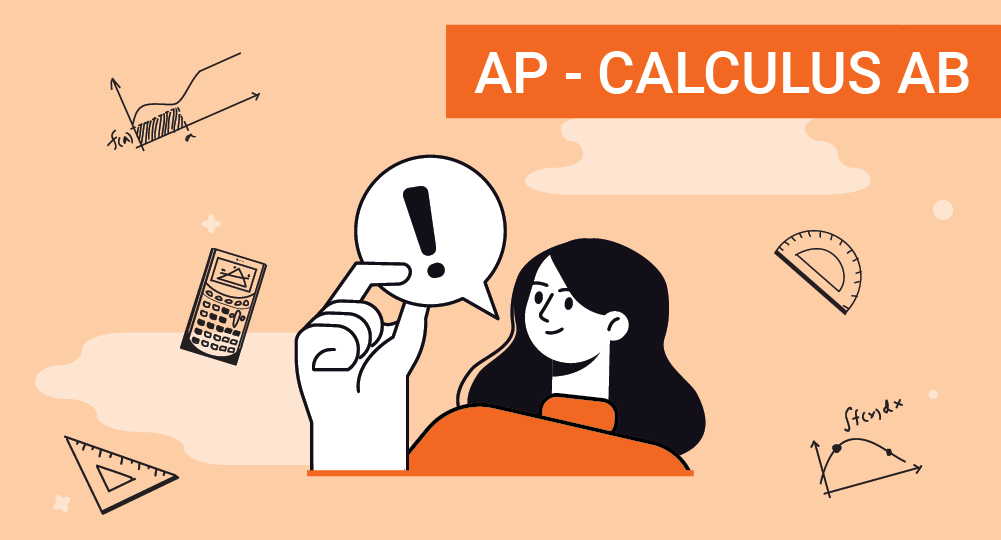#### Need Help?

Get in touch with usAP

# AP Calculus AB

Calculus AB

The AP Calculus exam, administered by the College Board, is a standardized test designed for high school students. Advanced Placement (AP) Calculus serves as an introductory college-level course in calculus. The exam evaluates students' comprehension and application of fundamental calculus concepts, including limits, derivatives and integrals. Through problem-solving and mathematical reasoning, students demonstrate their understanding of calculus techniques and their ability to analyze and solve real-world problems. The exam covers topics such as rates of change, optimization, differential equations, and applications of integration.

## Know more about AP Calculus AB

AP Calculus AB is an Advanced Placement course and exam offered by the College Board. It is designed to provide high school students with a college-level understanding of calculus and is typically taken by students who have completed a pre-calculus course. Calculus AB covers fundamental concepts in differential and integral calculus.

###### Course Overview:

AP Calculus AB covers fundamental concepts in differential and integral calculus.

It is typically taken by high school students who have completed a pre-calculus course.

Key Concepts:

• Limits and Continuity

• Derivatives and their applications

• Integrals and their applications

###### Exam Details:

The AP Calculus AB exam assesses students' understanding of calculus concepts and problem-solving skills.

• It consists of two main sections: multiple-choice questions and free-response questions.

• The multiple-choice section contains around 45 questions.

• The free-response section includes six questions

## Test Pattern

S No Test Section Number of Questions Time Allotted
1 Multiple Choice 45 105 minutes
2 Free Response 6 90 minutes

## What you'll learn

• 1

Limits and continuity: Understanding limits conceptually and algebraically, Evaluating limits graphically and numerically, Continuity and the Intermediate Value Theorem

• 2

Differentiation: Definition and Basic Derivative Rules, Derivatives of polynomial, exponential, logarithmic, and trigonometric functions

• 3

Differentiation: Chain rule and implicit differentiation, inverse trigonometric, Higher-order derivatives

• 4

Contexual applications of differentiation: Related rate, rate of change, linearization, L’Hospital’s Rule

• 5

Analytical Applications of Differentiation: Mean value theorem, Extreme value theorem, Concavity, Optimization, Curve sketching and analysis of functions

• 6

Integration and Accumulation of Change: Areas with Reimann sum, Definite integrals and the Fundamental Theorem of Calculus, Techniques of integration, including substitution and integration by parts

• 7

Differential equations: Modeling and verifying solutions, Slope fields and separation of variables, Exponential models, Euler's method

• 8

Applications of Integration: Accumulation functions and definite integrals, Finding the Area between curves, Finding volumes using different techniques

## Skills you'll learnIdentify an appropriate mathematical rule or procedure based on the classification of a given expressionExplain how an approximated value relates to the actual valueIdentify mathematical information from graphical, numerical, analytical, and/or verbal representationsDescribe the relationships among different representations of functions and their derivativesConfirm whether hypotheses or conditions of a selected definition, theorem, or test have been satisfiedExplain the meaning of mathematical solutions in contextIdentify an appropriate mathematical definition, theorem, or test to applyConfirm that solutions are accurate and appropriateUse precise mathematical language and appropriate units of measure and symbols